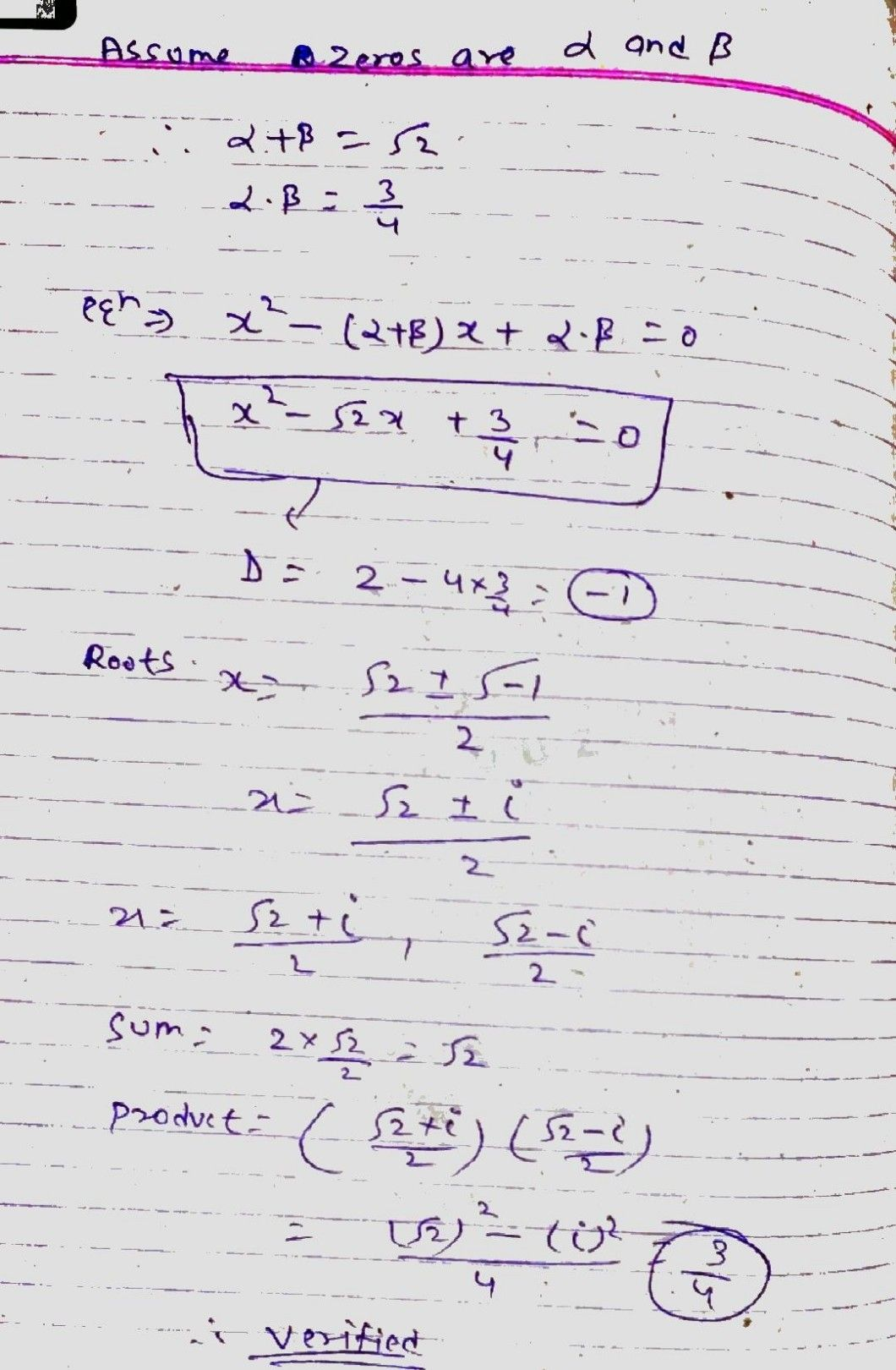Symbol
ProblemFind the lenguh the string, assuming that there $12$ Rd $1A$ $3$ in the strin $ci$ $An$ Answer the following questions. Each question carries three marks. $127\right)$ $38$ $1$ the sum and the product of the $zeroe9$ of quadratic polynomial $115\sqrt{2} and\dfrac {3} {4}$ then $t0\pi \pi$ the $\square$ polynomial and verify the relationship between coefficients and zeroes of polynomial. $4④$
10th-13th grade
Other
Search count: 107
SolutionQanda teacher - AskShivaniDear, Please evaluate and give GIFT coins(DOES NOT REDUCE YOUR COINS). 1. Click on top right corner EVALUATE button. 2. Select 5 star. 3. Click on gift coins + three times(C3000) 4. Click on DONE button on top right corner.
You can ask me more questions by clicking on my pic and then "Show more" after that click on "(1:1) ask a question".